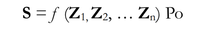# basic function for security and crisis situations: S = f(Z_1, Z_2, ..., Z_n) Po

#### humanistnoalgebra

##### New member
Hi, what this basic function mean? The article is about security and crisis situations (DOI: 10.5604/01.3001.0009.2966

The state of security is a function of many variables that we can present by means of the following formula:S: state of security, Z1, Z2, ... Zn – threat 1, threat 2, (...) threat n , Po : defence potential

sry haven't seen algebra in along time, writing mostly humanistic essays and articles (please speak to me like to a 4 y old), between the Po and the bracketts is a multiply or its just a name for the number of threats? if the number of threats increases the defence potential decreases, what is relation ? Where I can find more on similar functions?

#### Otis

##### Senior Member
Hello. The entire symbol $$\displaystyle f(Z_1, Z_2, Z_3, … Z_n)$$ is function notation, and it represents a single number. That number is called the 'function output'. This particular function has been named $$\displaystyle f$$. In function notation, the function's 'inputs' are listed inside the parentheses. The function's output depends upon its inputs; in other words, the value of symbol $$\displaystyle f(Z_1, Z_2, Z_3, … Z_n)$$ depends upon the numbers inside the parentheses.

Yes, your guess is correct. The number $$\displaystyle f(Z_1, Z_2, Z_3, … Z_n)$$ is multiplied by the number $$\displaystyle P_o$$, and that result is assigned to symbol $$\displaystyle S$$.

They have not provided a definition for function $$\displaystyle f$$, so even if somebody were to give you values for each threat level $$\displaystyle Z_i$$ and for the defense potential $$\displaystyle P_o$$ you would still not be able to calculate a value for $$\displaystyle S$$. In other words, the equation that they have provided is only a symbolic statement. Without the function definition or an example using actual numbers, we don't know what arithmetic they might be doing with their numbers $$\displaystyle Z_1, Z_2, Z_3, … Z_n$$ and $$\displaystyle P_o$$.

It could be that they have not actually determined a definition, yet. That equation might be a general statement that is intended only to express something like, "We would objectively quantify 'security state' as a function of multiple, subjective 'threat levels' and a 'defense potential' parameter", without actually having done it.

You can google keywords function notation, to find lessons, videos and examples of how the notation is defined and used. Cheers$$\displaystyle \;$$

Last edited:
•MarkFL

#### JeffM

##### Elite Member
What is intended exactly is unclear. Although mathematical notation is supposed to be above culture, it is not. Mathematical notation varies slightly between cultures (although far less than natural languages). In this case, there is what I can only view as a pretence at some kind of quantification.

However, all it merely says is that security is determined by the countervailing pressures of threats and defenses. (I do not believe the formula even fully captures that idea, but that seems to be what is intended.) To the extent that threats and defenses can approximately be measured quantitatively, then security can also be measured approximately. Of course, if no such numbers can be consistently validated over time, such claims seem to be no more than spurious rigor and any field dependent on such mathematical formalism is merely the homage that voodoo pays to arithmetic.